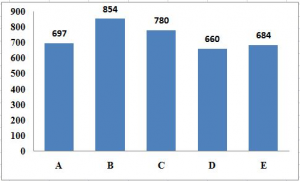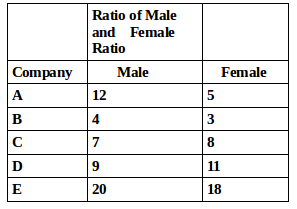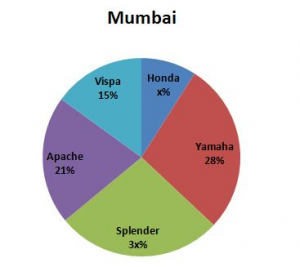## Data Interpretation For SBI PO : Set – 46

D.1-5) Study the following graph and table carefully to answer the given questions.
Number of employees working in 5 different Companies1) What is the total number of male employee in all the companies together ?

a) 2010

b) 2001

c) 2100

d) 2140

e) None of these

b)

A = 697*12/17 = 492

B = 854*4/7 = 488

C = 780*7/15 = 364

D = 660*9/20 = 297

E = 684*20/38 = 360

Total = 492+488+364+297+360 = 2001

2) What is the average number of female employee taking all the years together ?

a) 432

b) 278

c) 384

d) 335

e) None of these

d)

A = 697 – 492 = 205

B = 854 – 488 = 366

C = 780 – 364 = 416

D = 660 – 297 = 363

E = 684 – 360 = 324

Avg = 205+366+416+363+324/5 = 1674/5 = 334.8 = 335

3) What is the ratio of male and female employee working in companies D and E together ?

a) 217:221

b) 43:47

c) 124:127

d) 219:229

e) None of these

d)

Male = D+E = 297+360 = 657

Female = D+E = 363+324 = 687

657:687 = 219 : 229

4) By what % is the no of total employees of company C more than that of Company D ?

a) 18.18%

b) 16.75%

c) 22.65%

d) 7.25%

e) None of these

a)

C = 780

D = 660

% = (780-660)*100/660 = 18.18%

5) By what % is the no of employees working in companies A and C more than that of female employees working  in companies B and D ?

a) 112.5%

b) 96.2%

c) 102.6%

d) 124.5%

e) None of these

C)

A+C = 697+780 = 1477

Female B + D = 366+363 = 729

% = (1477-729)*100/729 = 102.6%

D.6-10) Study the following graph and table carefully to answer the given questions.
The pie-charts show the % of registration of different types of bikes in Mumbai and Delhi.Total no of bikes registered in Mumbai and Delhi is 15,000 and 350006) If no of Honda bikes registered in Mumbai is half of the % of  Vispa bikes registered in Delhi. Find the total number of Honda bikes registered in Mumbai and Delhi together ?

a) 5500

b) 5550

c) 6050

d) 4050

e) None of these

b)

Mumbai Honda = 18/2 = 9%

= 9*15000/100 = 1350

Delhi Honda = 12*35000/100 = 4200

Total = 1350+4200 = 5550

7) Find the no of Splender bikes registered in Delhi ?

a) 7700

b) 5100

c) 6500

d) 4700

e) None of these

a)

Delhi Splender = 100 –(18+12+25+23)

= 100 – 78 = 22%

12% = 22*35000/100 = 7700

8) Which of the following bikes registration has the maximum difference between Delhi and Mumbai ?

a) Splender

b) Yamaha

c) Apache

d) Vispa

e) None of these

c)

Mumbai          Delhi

Honda             9% = 1350     12% = 4200

Yamaha          28%=4200      25% = 8750

Splender         27%=4050      22% = 7700

Apache           21%=3150      23% = 8050

Vispa              15%=2250      18% = 6300

8050 – 3150 = 4900….Apache

9) What is the ratio of no of Vispa bikes registered in Mumbai to the no of Yamaha bikes registered in Delhi ?

a) 11:26

b) 24:7

c) 35:8

d) 9:35

e) None of these

d)

V : Y = 2250 : 8750 = 225 : 875 = 9:35

10) Which of the following bikes registered maximum in Mumbai ?

a) Apache

b) Honda

c) Yamaha

d) Splender

e) None of these